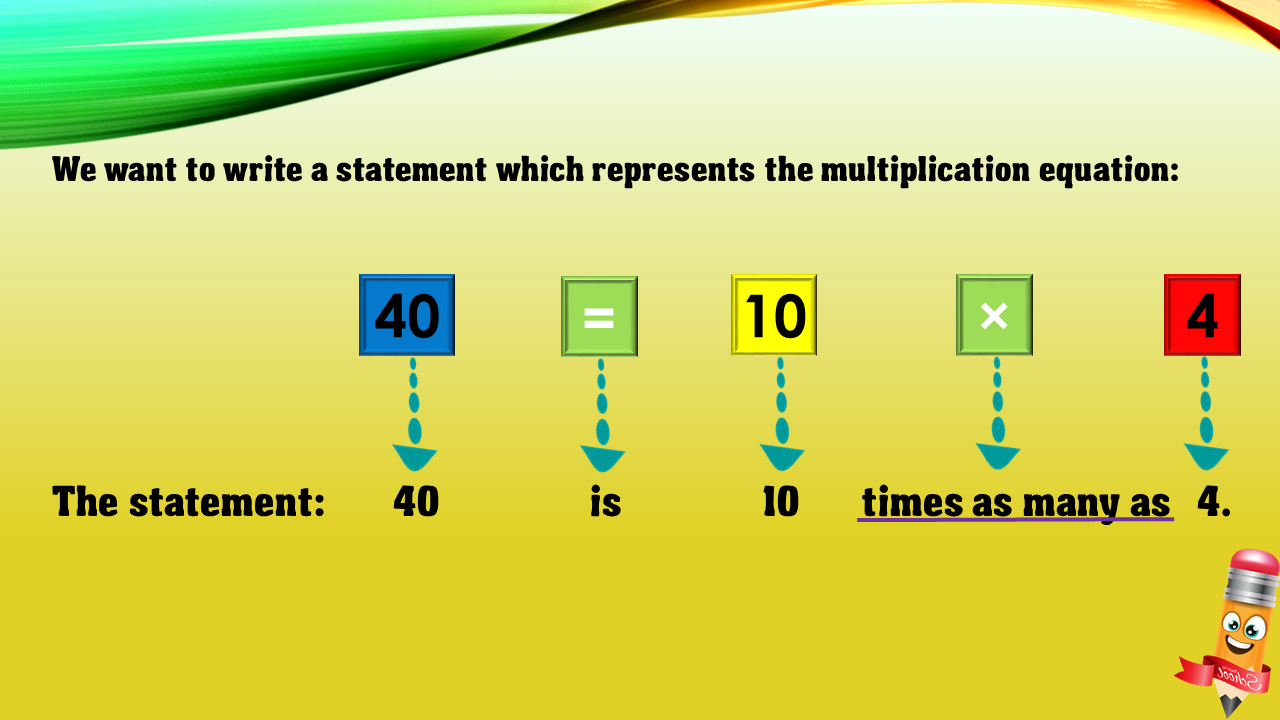1
visibility

Choose one statement which represents the equation: 40 = 10×4 ?

• A

40 times as many as 10 is 4.

• B

40 is 10 more than 4.

• C

40 is 10 times as many as 4.

• D

10 is 4 less than 40.